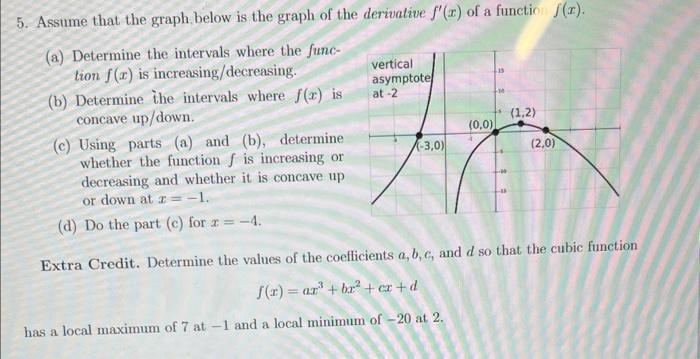# (Solved): 5. Assume that the graph below is the graph of the derivative f(x) of a function f(x). (a) Deter ...5. Assume that the graph below is the graph of the derivative of a function . (a) Determine the intervals where the function is increasing/decreasing. (b) Determine the intervals where is concave up/down. (c) Using parts (a) and (b), determine whether the function is increasing or decreasing and whether it is concave up or down at . (d) Do the part (c) for . Extra Credit. Determine the values of the coefficients , and so that the cubic function has a local maximum of 7 at -1 and a local minimum of -20 at 2 .

We have an Answer from Expert